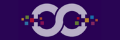# Simplifying The Greeks: Theta (Part of a Series)

#1

By Darrell Martin

Continuing with defining the Greeks in simple to understand, English terms, this article will explain Theta. Remember, Greeks are merely a fancy way to say a math formula to measure or explain a price movement.

Theta is the eighth letter of the Greek alphabet. This is how it is written in upper Θ and lowercase θ. When used in finance, the uppercase form is used.

Theta is how much the price of the option will change with time decay. This is actually quite useless when trading spreads. Why? Because it is a measurement of one day’s time value. There is only one day or less in all Nadex spreads.

• Theta is lost when an option is Bought.

• Theta is made when an option is Sold.

In reality, all that needs to be known when trading spreads is the Premium, which is the extrinsic value, or the price minus where the underlying market is currently trading. When trading Nadex spreads, use the Indicative Index as the reference for the current underlying market.

Look at this example on Wall St 30. The following example shows two spreads with the same ranges (floors and ceilings) but different expirations.

This image shows how Theta (Premium) decays with time. Notice the bid price of the 17775-17875 spread expiring at 4:00PM. It is 17819. When the Indicative Index of 17817 is subtracted from it, the premium is +\$2, which is Theta. Contrast this with the offer price of the same spread: 17823 minus 17817 is \$6, but since the offer price is above the Indicative, premium would be reflected as a negative number.

Keep this simple since Theta is the one-day time value of a spread. All of the extrinsic value is Theta; there is no hard math or graphs needed.

Look at the following things: Where is the underlying market quote? Where is the spread’s price? The further away they are from each other, the slower the contract will move. It does not matter if it is above it or below it. The “proximity” will determine the “speed” of the spread’s price change relative to the price change of the underlying market.

The following example may help in understanding how Theta works when trading instruments held longer than one day.

Suppose that Theta is .03 and an option is bought. It would lose .03 a day in extrinsic value. It may gain or lose more from movement of the underlying or if volatility changes.

Theta is on a curve. For example: .03 three hours away .04 two hours away .05 one hour away

Then Theta will rise rapidly (as all extrinsic value has to be gone before expiration.) If the option is At the Money, or Out of The Money, then it would lose value much faster. Theta drops over time, not just in the last five minutes. There are no complex graphs needed. Simply consult a price ladder.

Theta can be overridden by implied volatility (IV). This means premium can rise as expiration approaches, if IV rises.

Remember, Theta is the amount the option will make or lose each day until expiration. It is very easy to figure on spreads that expire in one day or less.